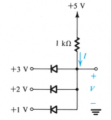# Difficulty Analyzing Simple Ideal Diode Circuit (AND Gate)

#### ElectronicGuru

Joined Sep 26, 2014
22
Here's the circuit for which I must find I and V:I know that with ideal diode circuits, you have to "guess" whether the diode is forward or reverse biased (short or open circuit, respectively).

I'm looking at this circuit above and am getting nowhere with the analysis. The tricky thing for me is those 3 voltages at the bottom: If I assume that all three diodes are on (short circuit), then how do I determine V? Clearly, I'm forgetting some basic circuit analysis here.

#### WBahn

Joined Mar 31, 2012
26,487
You pretty much have to "guess" the state of each diode whether it is ideal or not.

With practice, you'll be able to look at a circuit like this and tell by inspection which diodes are on and which are off. In the meantime, you can always rely on basic principles. You have, at most, eight possible states to consider. For each state, if a diode is ON then it has zero voltage across it (and the current through it must be zero or more, i.e., positive) while if it is OFF the voltage across it must be zero or less, i.e., negative, (and the current through it must be zero). The voltage is the voltage of the anode relative to the cathode and the current is the current entering the anode. If the resulting currents and voltages are not self-consistent, then you know that that set of guesses states is wrong.

For instance, if you guess that two diodes are on but that requires that the voltage on a particular node have two different values, then you know that those two diodes are not both on. Apply this rule mentally to the above circuit and you can eliminate half of the possible states immediately.

•ElectronicGuru

#### MikeML

Joined Oct 2, 2009
5,444
Think about where the current flows. The current flows from most positive node to the most negative node in the circuit.

Assume that when a diode conducts, it's forward voltage is 0.6V. Based on that assumption, what is the voltage at V+?

#### crutschow

Joined Mar 14, 2008
30,117
If you want to calculate the values and not try to guess which diodes are forward biased just do the voltage calculation for one diode at a time with the other two diodes removed.
After you determine these three voltages you will be able to see which are forward biased and which are reverse biased when all the diodes are in the circuit.

#### WBahn

Joined Mar 31, 2012
26,487
If you want to calculate the values and not try to guess which diodes are forward biased just do the voltage calculation for one diode at a time with the other two diodes removed.
After you determine these three voltages you will be able to see which are forward biased and which are reverse biased when all the diodes are in the circuit.
But realize that doing it this way IS just guessing. By doing the calculation for one diode with the others removed, you are simply guessing that the other two diodes are off. In this case that will get you the answer because only one of the diodes is on. But in general you might have more than one diode on and so this approach only gets you part way and you need to be able to ascertain which part it has gotten.